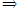# Describe the permutation function

Publish On: 2019-03-22

Total Post: 542

# Mjay Jollay

Total Post: 0

## ANS: Describe the permutation function

A one-one function whose domain and the range is the same set, the set being finite, is called a permutation function. For example, let S = {1, 2, 3} be a finite set, then there are 3 ! = 6 permutation functions p1, p2, p3, p4, p5, p6 defined from S to S. Let us explain the permutation functions by means of the above table.

Inversion

Let p be a permutation function and i < j be a pair of elements in its domain such that p (i) > p (j), then p is said to have an inversion. For example if S = {1, 2} and the permutation function is p2, then we notice from the above table that 2 < ip(2) > p(1) and as such p2 has one inversion. Obviously, p1 has zero inversion. In other words, an inversion is said to take place if in a permutation 3, 1 and 2, we have the couples (1, 2) which gives one inversion.